## CHAPTER VIII THE CUBE GROUP B

THE members of this group are all cubes. They occur on the right hand swing of the pendulum. Their characteristic valence is three but they often show higher valencies. They all have six funnels, as in Cube Group A, but they show quite a different design in the arrangement of the Anu.

 ATOMIC No. ANU ELEMENT CENTRE 6 FUNNELS 13 486 Aluminium - 6 [Al.9' + 8 Al.9] 15 558 Phosphorus - 6 [(B5 + 3N6 + 3P9) + (Li4 + 3Be4 + 3P9)] 31 1260 Gallium - 6 [(Ga7 + 3Ga15 + 3Ga20) + (B5 + 3Ga13 + 3Ga18)] 33 1350 Arsenic - 6 [Al.9' + 8 (2N9 + Al.9)] 49 2052 Indium - 3 [2 (In16 + 3Ga15 + 3Ga20) + (In14 + 3Ga13 + 3Ga18)] + 3 [(In16 + 3Ga15 + 3Ga20) + 2(In14 + 3Ga13 + 3Ga18)] 51 2169 Antimony - 3 [2Sb128 + Sb113] + 3 [Sb128 + 2Sb113] 64 2880 Gadolinium Ne120 3 [2Sb128 + Sb113 + (Ca45 + 2N24)] + 3 [Sb128 + 2Sb113 + (Ca45 + Mo11 + 2N24)] 66 2979 Dysprosium Ne120 3 [2Sb128 + Sb113 + (Ca45 + 2Mo11 + 2N24)] 3 [Sb128 + 2Sb113 + (Ca45 + 2Mo11 + 2N24)] 81 3678 Thallium Tl.687 3 [2Sb128 + Sb113 + (Ca45 + Ti.44 + 2N24)] + 3 [Sb128 + 2Sb113 + (Ca45 + Ti.44 + 2N24)] 83 3753 Bismuth Tl.687 3 [2Sb128 + Sb113 + (Ca45 + Mo46 + 2N24)] + 3 [Sb128 + 2Sb113 + {Ti88 + (Ga20 + 4Zr13)}]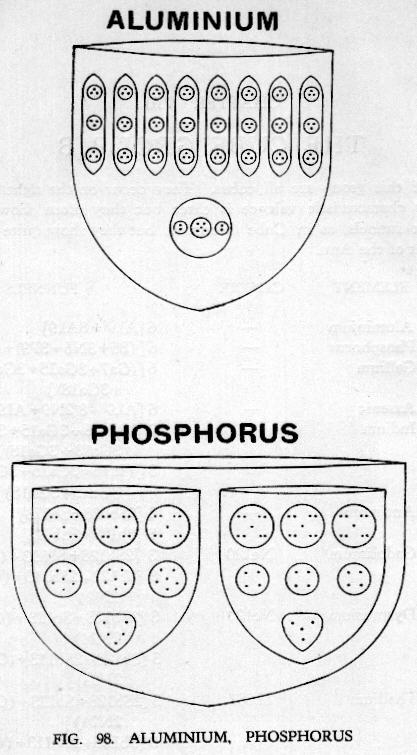FIG. 98. ALUMINIUM. PHOSPHORUS

• ATOMIC No. 13
• ALUMINIUM
• As the head of the group this element is, as usual, simple. There is no central globe. Fig. 98.
• Funnels. The six funnels each contain eight similar ovoids, Al.9, of three spheres of three Anu. Below these is an ovoid Al.9', again of three spheres, these being two duads and a pentad.
• Aluminium = 6 (Al.9' + 8 Al.9)
• 6 funnels of 81 Anu = 486 Anu
• Total = 486 Anu
• Number weight 486 / 18 = 27.00tk

• ATOMIC No. 15
• PHOSPHORUS
• Like Aluminium, Phosphorus has no central globe. Fig. 98.
• Funnels. Each of the six funnels contains two segments.
• Segment A contains at the bottom a group 135, then three N6, and then three spheres, P9, of nine Anu, making 50 Anu in all. Segment B also contains seven spheres, first a tetrad Li4, with its Anu at the corners of a tetrahedron, then three spheres of four Anu, Be4, and then the three spheres, P9, of nine Anu found in segment A. Segment B contains 43 Anu.
• Phosphorus = 6 [(B5 + 3N6 + 3P9) + (Li4 + 3Be4 + 3P9)]
• 6 funnels of 93 Anu = 558 Anu
• Total = 558 Anu
• Number weight 558 / 18 = 31.00tk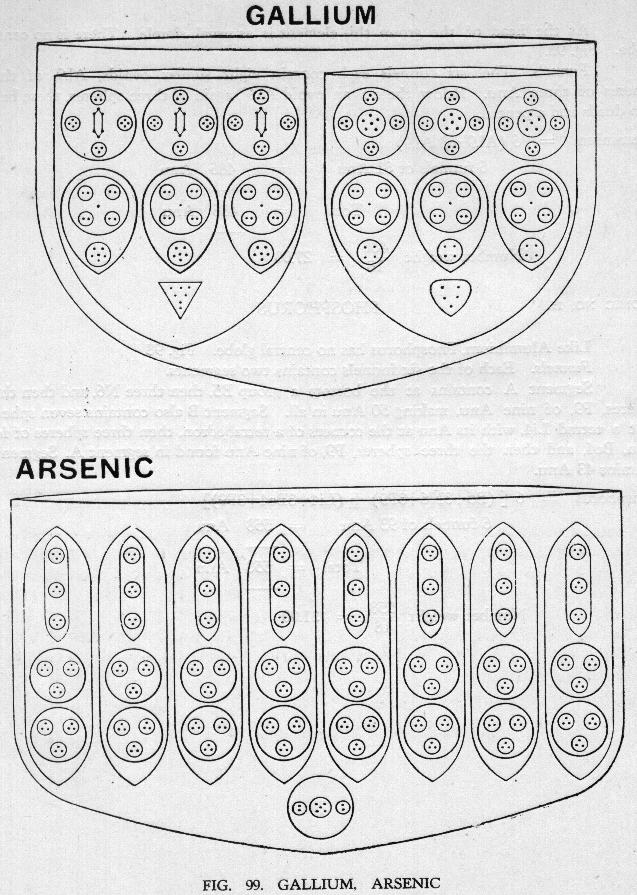FIG. 99. GALLIUM. ARSENIC

• ATOMIC No. 31
• GALLIUM
• There is no central globe in this element. Fig. 99.
• Funnels. Gallium has six similar funnels, each with two segments. Segment A. There is first a cone of 7 Anu, then three rather curiously formed groups of fifteen Anu, containing a P9 group and an N6. The three upper spheres, Ga20, in segment A are made up of two Be4 and two H3 in the form of a cross with an Ad6 in the centre. The total number of Anu in segment A is 112 Anu. Segment B is somewhat similar to segment A. It contains first a B5 instead of the cone, then three groups Ga13, made up of P9 and Be4, and then a row of three spheres Ga18, each with four H3 in the form of a cross with N6 in the centre. Segment B contains 98 Anu.
• Gallium = 6 [(Ga7 + 3Ga15 + 3Ga20) + (B5 + 3Ga13 + 3Ga18)]
• 6 funnels of 210 Anu = 1260 Anu
• Total = 1260 Anu
• Number weight 1260 / 18 = 70.00tk

• ATOMIC No. 33
• ARSENIC
• Once more there is no central globe. Fig. 99.
• Funnels. All six funnels are alike, and there are not two separate segments. Arsenic resembles Aluminium in having eight internal sub-divisions in the funnels. and the ovoids which form the top ring are identical with those in Aluminium save for the minute difference that in Aluminium the ovoids stand the reverse way from those in Arsenic. In Arsenic the top and bottom triplets in the top ovoids point downwards and the middle one upwards, in Aluminium the opposite is true. Arsenic inserts sixteen spheres, in two rows of eight, between the ovoids and the bottom globe Al.9', which is similar to that in Aluminium. Each of these 16 spheres contain nine Anu, N9, so that Arsenic adds no less than 144 Anu to each funnel of Aluminium. The total in one Arsenic funnel is 225 Anu.
• Arsenic = 6 [Al.9' + 8 (2N9 + Al.9)]
• 6 funnels of 225 Anu = 1350 Anu
• Total = 1350 Anu
• Number weight 1350 / 18 = 75.00tk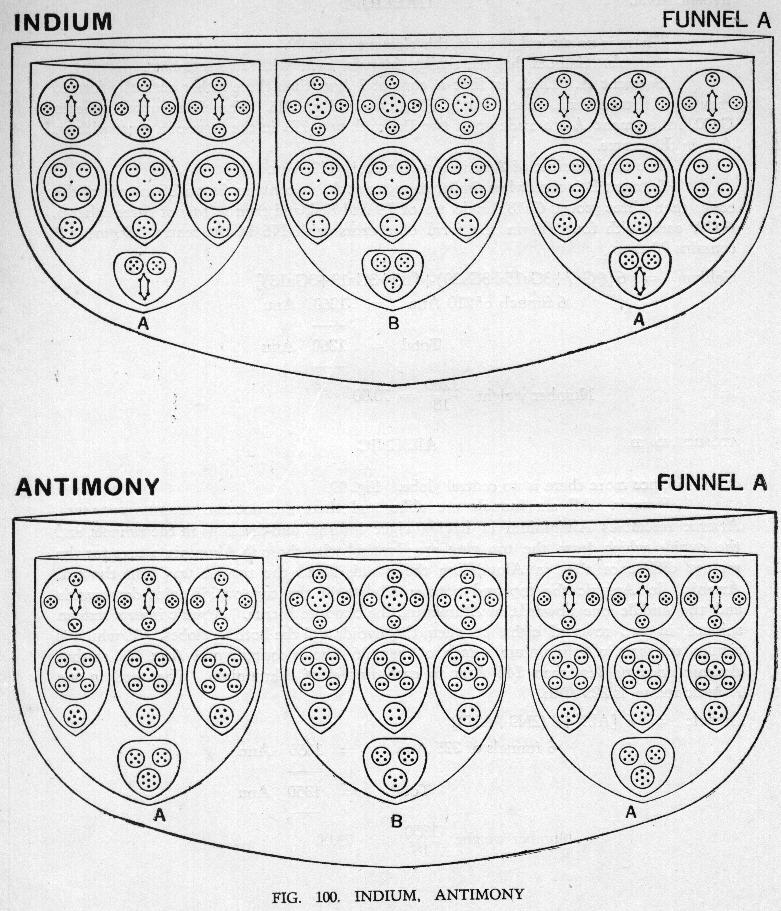FIG. 100. INDIUM, ANTIMONY

• ATOMIC No. 49
• INDIUM
• This element also has no central globe. Fig. 100.
• Funnels. Each of the six funnels has three segments instead of two, and the segments are of two types. Three funnels contain two segments of type A and one of type B; the other three funnels contain two segments of type B and one of type A. Segment A is similar to segment A of Gallium, save in the substitution of a body of 16 Anu for the cone of 7 Anu, making a total of 121 Anu. Segment B is similar to the B segment of Gallium save that a body of 14 Anu. In14, is substituted for the B5 group, making a total of 107 Anu.
• Type A funnels contain 2A + B. Type B funnels contain A + 2B.
• Indium = 3 [2 (In16 + 3Ga15 + 3Ga20) + (In14 + 3Ga13 + 3Ga18)] + 3 [(In16 + 3Ga15 + 3Ga20) + 2 (In14 + 3Ga13 + 3Ga18)]
• Three funnels 2A + B = 1047 Anu
• Three funnels A + 2B = 1005 Anu
• Total = 2052 Anu
• Number weight 2052 / 18 = 114.00tk

• ATOMIC No. 51
• ANTIMONY
• This element is very similar to Indium. There is no central globe. Fig. 100.
• Funnels. As in Indium there are two types of funnels and each funnel contains three segments, of types A and B.
• Segment A consists of seven bodies. Nearest the centre we find a group containing two quintets and a septet I.7. This group may be identified as Sb17. Then comes a ring of three spheres containing 17 Anu, Sb17. In this sphere we find that the unit in the centre of the P9 group has been replaced by a triplet. Finally there is another ring of three globes each containing two triplets, two quartets and an Ad6. This is Ga20. This whole segment is Sb128. Segment B also contains seven bodies, first a group of three small spheres which is identical with In14, then a ring of three Sb15, and finally three Ga18, the whole making Sb113.
• So in Antimony we find three funnels of Type A containing 2A + B segments and three funnels of Type B containing A + 2B.
• Antimony = 3 (2Sb128 + Sb113) + 3 (Sb128 + 2Sb113)
• 3 funnels 2A + B = 1107 Anu
• 3 funnels A + 2B = 1062 Anu
• Total = 2169 Anu
• Number weight 2169 / 18 = 120.50tk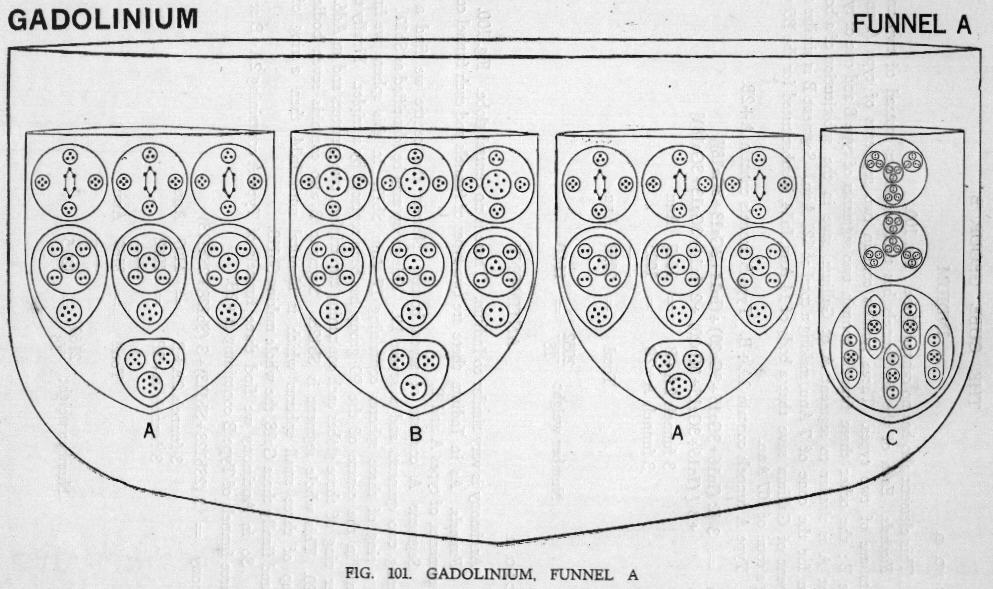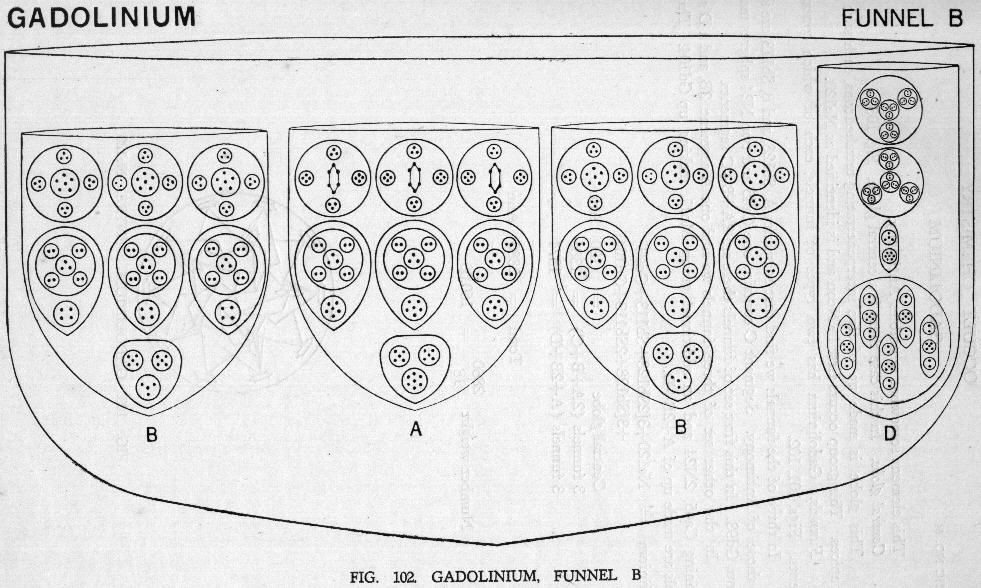• ATOMIC No. 64
• This element somewhat resembles Antimony.
• Central globe. In this case there is a central globe. Fig. 103.
• This globe is made up of five interpenetrating tetrahedrons, each with an Ad6 at its apices. This group occurs first in Neon and is identified as Ne120.
• Funnels. Gadolinium has two types of funnels, each of which contains four segments. Figs. 101, 102.
• In three of the funnels we find that segments A, Sb128, and B, Sb113, are identical with those of Antimony. Segment C contains one Ca45 and two N24 spheres making up 93 Anu, Gd93. In this first set of funnels there are 2A + B + C segments.
• In the other set of three funnels we find one A segment, 213, and a D segment
• containing Ca45, 2N24 and an additional group Mo11, making up Gd104. Thus these
• tunnels are made up of A + 2B + D.
• Gadolinium = Ne120 + 3 [2Sb128 + Sb113 + Gd93] + 3[Sb128 + 2Sb113 + Gd104]
• Central globe = 120 Anu
• Three funnels (2A + B + C) = 1386
• Three funnels (A + 2B + D) = 1374
• Total = 2880 Anu
• Number weight 2880 / 18 = 160.00tk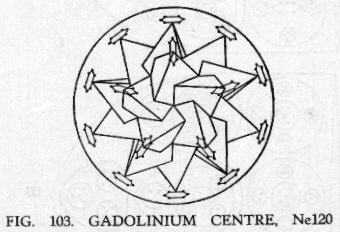• ATOMIC No. 66
• DYSPROSIUM
• This element much resembles Gadolinium.
• Central globe. The central globe is formed of the five interpenetrating tetrahedrons as in Gadolinium, Ne120. Fig. 104.
• Funnels. The six funnels each contain four segments and these are of three types. Segment A is Sb128, Segment B is Sb113, Segment C is Gd93 with the addition of two Mo11 groups, making Ds115. Figs. 105, 106.
• Three funnels are composed of 2A + B + C and the other three of A + 2B + C.
• Dysprosium = Ne120 + 3[2Sb128 + Sb113 + Ds115] + 3[Sb128 + 2Sb113 + Ds115]
• Central globe = 120 Anu
• 3 funnels each 2A + B + C = 1452
• 3 funnels each A + 2B + C = 1407
• Total = 2979 Anu
• Number weight 2979 / 18 = 165.50tk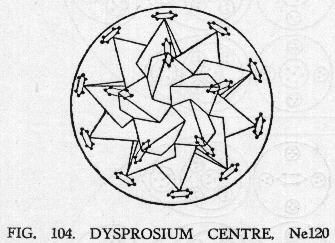FIG. 104. DYSPROSIUM CENTRE. Ne120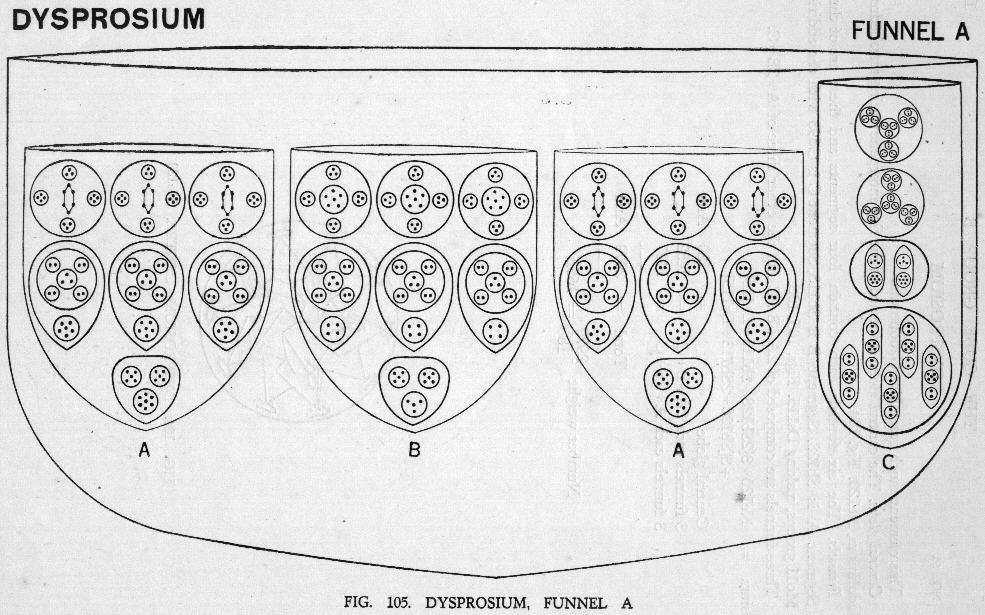FIG. 105. DYSPROSIUM, Funnel A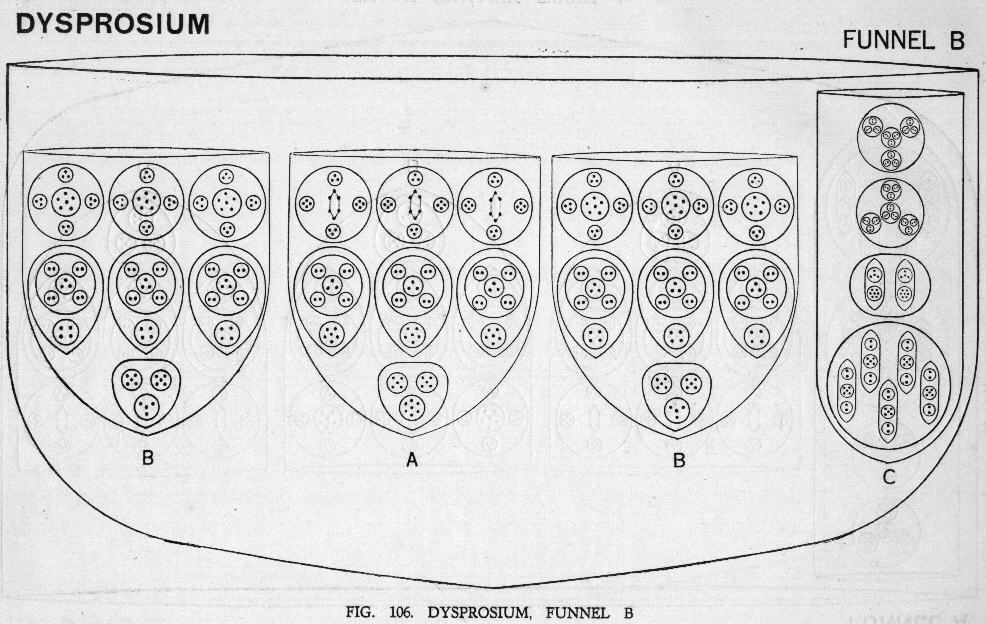FIG. 106. DYSPROSIUM, Funnel B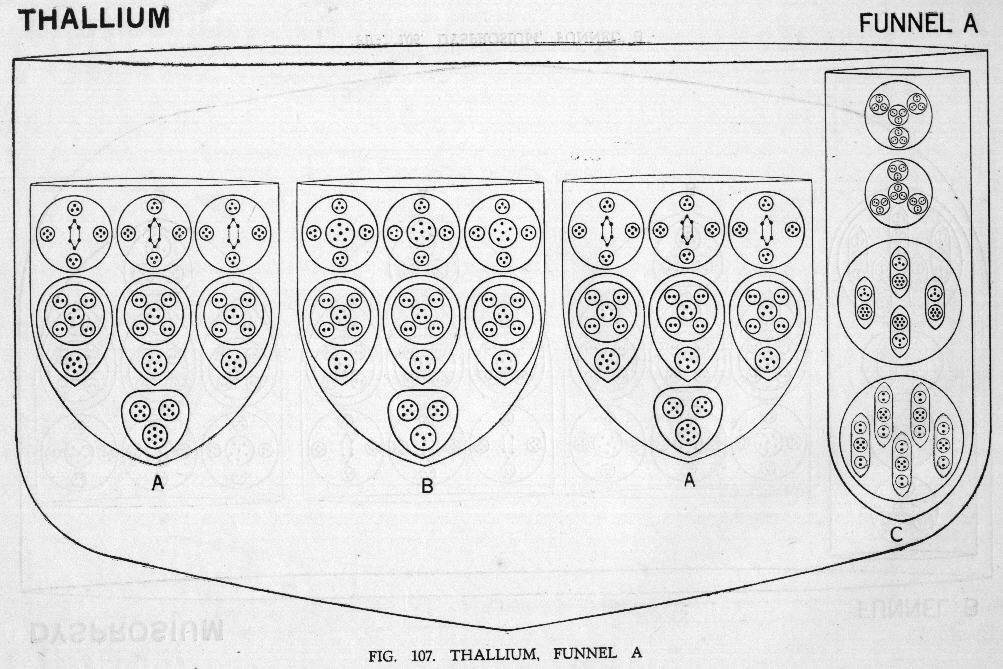FIG. 107. Thalluum, Funnel A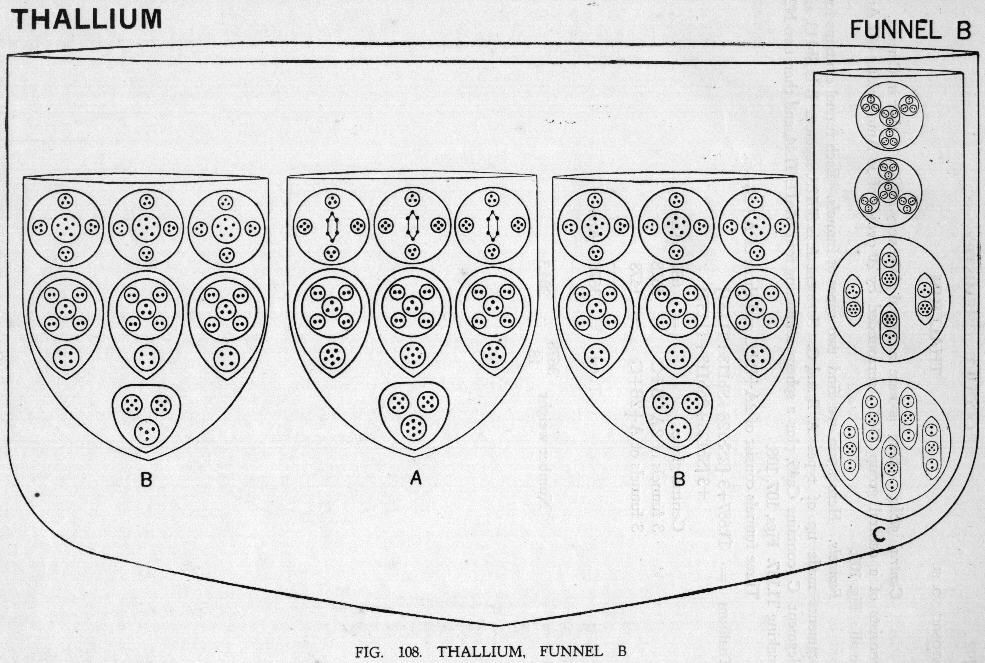FIG. 108. Thalluum, Funnel B

• ATOMIC No. 81
• THALLIUM
• Central globe. There is here a central globe very similar to that in Cerium. It consists of a central group, Ce27, surrounded by 20 ovoids of 33 Anu, making 687 Anu in all. Fig. 109.
• Funnels. Here again we find two types of funnels. Each funnel contains four segments made up of types A, B and C. Segment A is Sb128, segment B is Sb113, and Segment C contains Ca45, plus a sphere containing four Mo11, Ti.44, and then two N24, making Ti.137. Figs. 107, 108. Three funnels consist of 2A + B + C and three of A + 2B + C.
• Thallium = Tl.687 + 3 [2Sb128 + Sb113 + Ti.137] + 3 [Sb128 + 2Sb113 + Ti.137]
• Central globe = 687 Anu
• 3 funnels of 2A + B + C = 1518 Anu
• 3 funnels of A + 2B + C = 1473 Anu
• Total = 3678 Anu
• Number weight 3678 / 18 = 204.33tk

• ATOMIC No. 83
• BISMUTH
• Central globe. The central globe is similar to that of Thallium, Tl.687. Fig. 109.
• Funnels. Here again there are two types of funnels, each containing four segments, made up of types A, B, C and D.
• Segment A is Sb128,
• Segment B is Sb113.
• Segment C is composed of Ca45, Mo46 and two N24 groups making 139 Anu.
• Segment D is part of the arm of Zirconium and contains 160 Anu.
Three of the funnels contain 2A + B + C and three A + 2B + D. Figs. 110, 111.
• Bismuth = Tl.687 + 3 [2Sb128 + Sb113 + (Ca45 + Mo46 + 2N24)] + 3 [Sb128 + 2Sb113 + {Ti88 + (Ga20 + 4Zr13)}]
• Central globe = 687 Anu
• 3 funnels 2A + B + C = 1524
• 3 funnels A + 2B + D = 1542
• Total = 3753 Anu
• Number weight 3753 / 18 = 208.50tk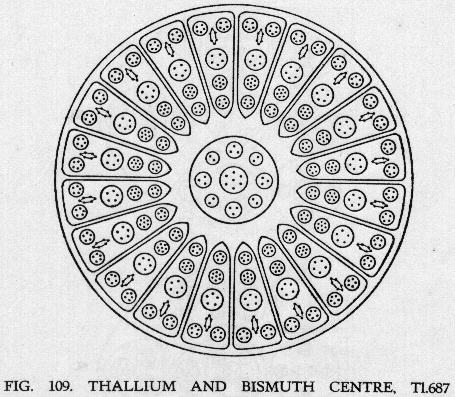FIG. 109. THALLIUM AND BISMUTH CENTRE, Tl.687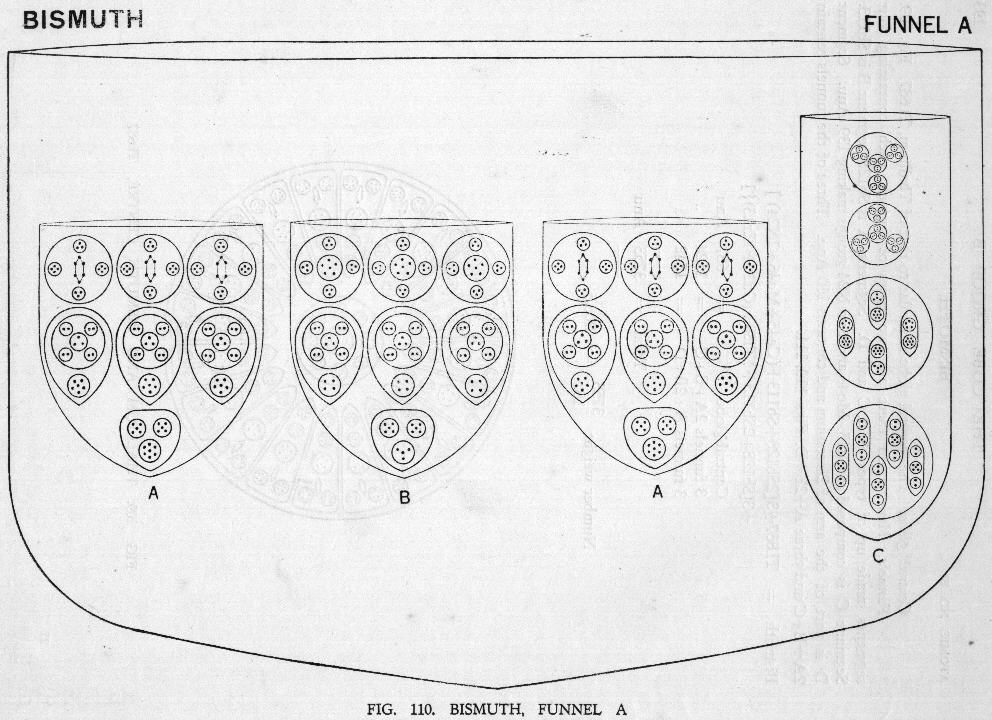FIG. 110. Bismuth, Funnel A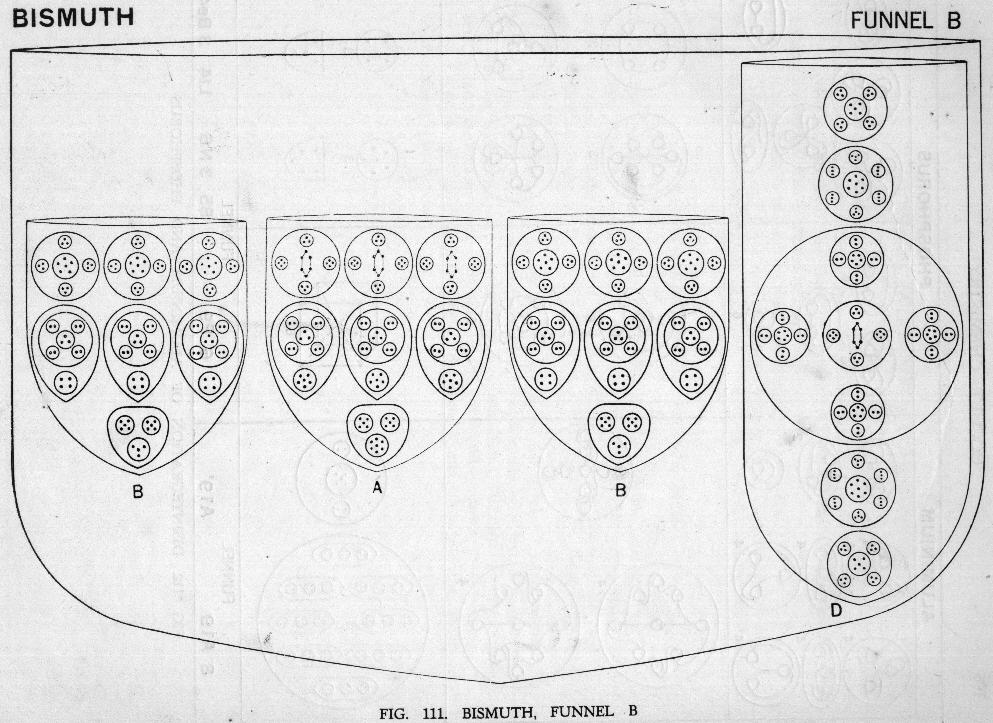FIG. 111. Bismuth, Funnel B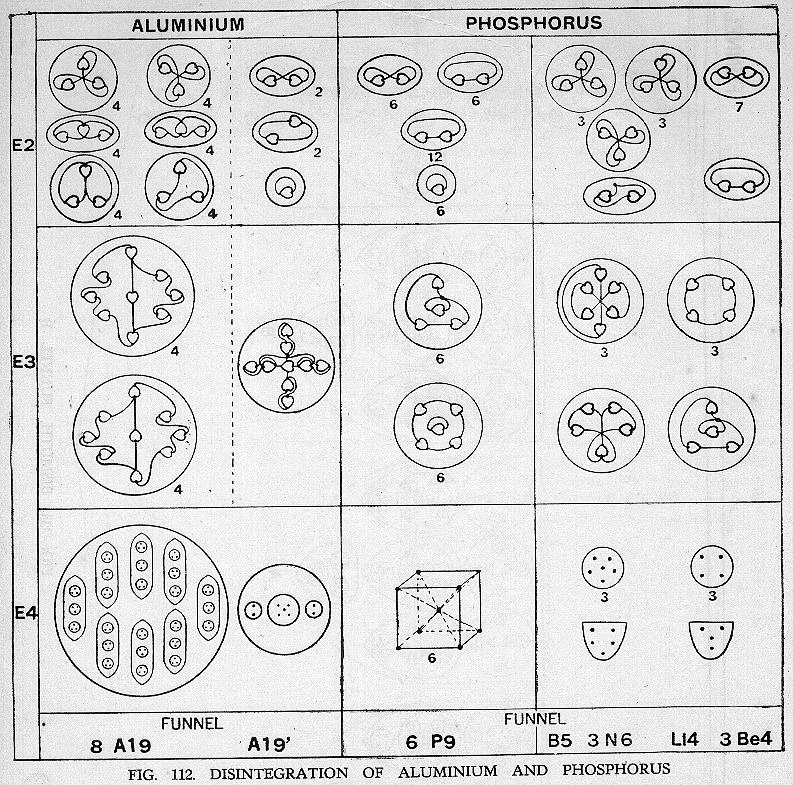FIG. 112. DISINTEGRATION OF ALUMINIUMD and PHOSPHORUS

### DISINTEGRATION OF CUBE GROUP B

• DISINTEGRATION OF ALUMINIUM
• The funnels separate and the contents are liberated. The eight ovoids remain together in a sphere, so two bodies from each funnel are set free on the E4 level. Fig. 112.
• On the E3 level the eight ovoids are set free and become spherical, forming bodies containing 9 Anu as shown in Fig. 112.
• On the E2 level each of these breaks up into three triads.
• The globe from the funnel becomes a cross at the E3 stage, with one Anu from the duads in each arm in addition to its own. On the E2 level these form four duads and a unit.
• DISINTEGRATION OF PHOSPHORUS
• On the E4 level segment A sets free three P9 groups, three sestets, N6, and a quintet, B5. The P9 groups form a cube with one Anu at each corner and one in the centre attached to all the others. Fig. 112.
• Similarly segment B, on the E4 level, liberates three P9, three quartets, Be4, and one quartet, Li4.
• On the E3 level the P9 groups each form two bodies. Five of the nine Anu hold together and place themselves on the angles of a square-based pyramid; the remaining four set themselves on the angles of a tetrahedron. The other groups form three sextets, and a quintet and three ring quartets and a pyramid as shown.
• On the E2 level each P9 yields 4 duads and a unit, while the other groups form triads and duads as shown.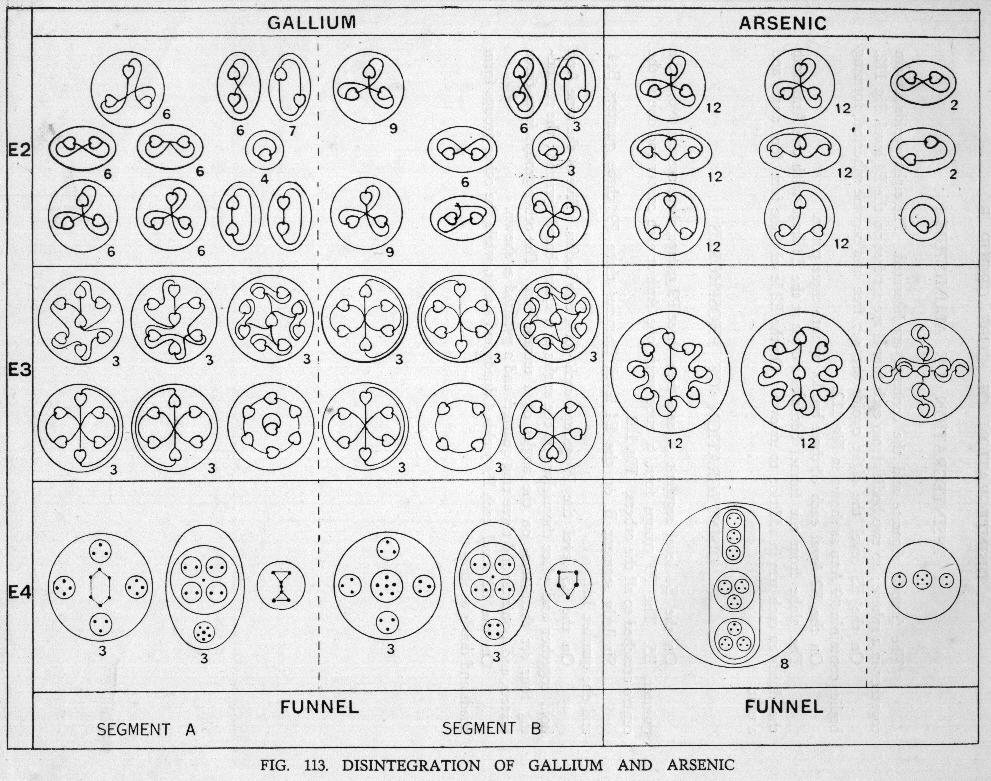FIG. 113. Disintegration of Gallium and Arsenic

• DISINTEGRATION OF GALLIUM
• In Gallium the funnels are liberated and then set free their two containing segments, each of which forms a cylinder. Thus each funnel yields two bodies at the first stage of the E4 level. This is not shown in Fig. 113. At the second stage the segments liberate their contents, each giving seven groups. Fig. 113.
• Segment A. On the E4 level this gives the three Ga20, three Ga15 and the small group of 7 Anu, Ga7.
• On the E3 level each Ga20 forms a sextet and two septets, the quartet and triad uniting. Each Ga15 forms a sextet and a cross with nine Anu having a duad in each arm and one Anu in the centre. The Ga7 forms a ring of six Anu with one in the centre.
• On the E2 level these all break up into triads, duads and units.
• Segment B. On the E4 level we find three Ga18, three Ga13 and a B5. On the E3 level each Ga18 gives three sextets, each Ga13 gives the cross of nine Anu as before and a ring quartet, and the B5 gives a quintet.
• On the E2 level these act as usual, giving triads, duads and units.
• DISINTEGRATION OF ARSENIC
• Arsenic resembles Aluminium in having eight ovoids in its funnel. These are set free as spheres on the E4 level, as is the globe of nine Anu, Al.9'. Thus we have nine spheres on this E4 level. Fig. 113.
• On the E3 level the three groups of nine Anu from the ovoids are liberated and form groups having the same design as those in Aluminium. The globe Al.9' gives a cross of nine Anu. On the E2 level triads, duads and units are formed at shown in Fig. 113.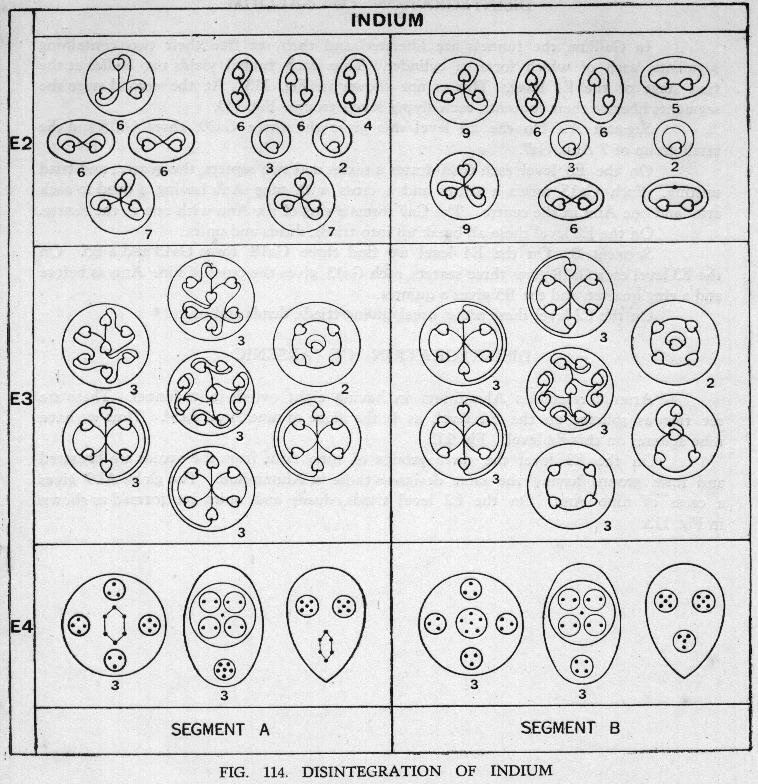FIG. 114. Disintegration of Indium

• DISINTEGRATION OF INDIUM
• After the funnels of Indium separate they set free their segments and these in turn liberate their contents. Each segment gives seven bodies. Each funnel contains three segments, these being of two types. A and B. Fig. 114.
• Type A.
• On the E4 level type A gives three Ga20, three Ga15 and an In16.
• On the E3 level each Ga20 gives a sextet and two septets as before. The Ga15 also acts as in Gallium giving a sextet and a cross of nine Anu. The In16 gives a sextet and two quintets formed of a ring of four Anu with one in the centre (a squarebased pyramid).
• Type B.
• On the E4 level we have three Ga18, three Ga13 and an In14.
• On the E3 level each Ga18 gives three sextets and the Ga13 gives the cross of nine Anu and a ring quartet as in Gallium. The In14 gives a tetrahedron quartet and two quintets (square-based pyramids).
• On the E2 level they give triads, duads and units as before.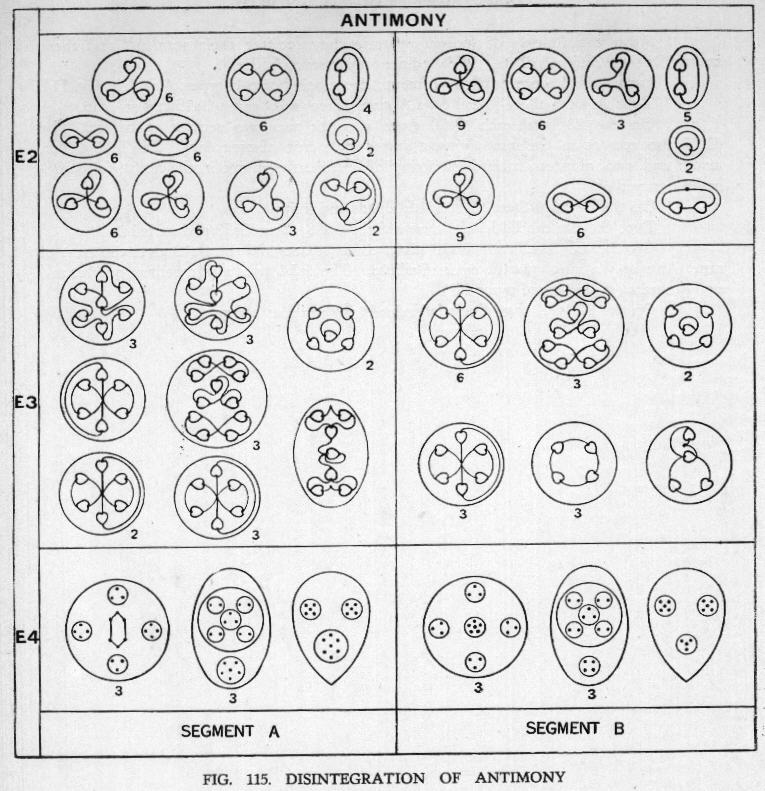FIG. 115. THE CUBE GROUP B

• DISINTEGRATION OF ANTIMONY
• This element follows Gallium and Indium in its disintegration. There are three segments in each funnel and these segments are of two types. Each liberates seven bodies on the E4 level. Fig. 115.
• Type A.
• On the E4 level we find three Ga20, three Sb17 and one Sb17'. On the E3 level each Ga20 gives a sextet and two septets. The Sb17 is like the Ga15 except that a triplet is substituted for the unit in the centre of the P9 group. This apparently throws the cross out of gear for we have a new figure of eleven Anu containing two quartets and a triplet. In addition to the body of eleven Anu each Sb17 liberates a sextet on the E3 level. The Sb17' gives a septet and two quintets of the square-based pyramid type.
• On the E2 level we find quartets, triplets, duads and units.
• Type B.
• On the E4 level we find three Ga18, three Sb15 and one In14.
• On the E3 level each Ga18 gives three sextets, the Sb15 is similar to Ga13 except for the substitution of a triplet for the unit in the centre. Each Sb15 gives the group of eleven Anu as in the A type segment and a ring of four Anu. The In14 gives a tetrahedron and two quintets of the square-based pyramid form.
• On the E2 level we find quartets, triplets, duads and units.

Fig. 116 shows the Cube Group B in a condensed form, from which the relationships in the group can be studied.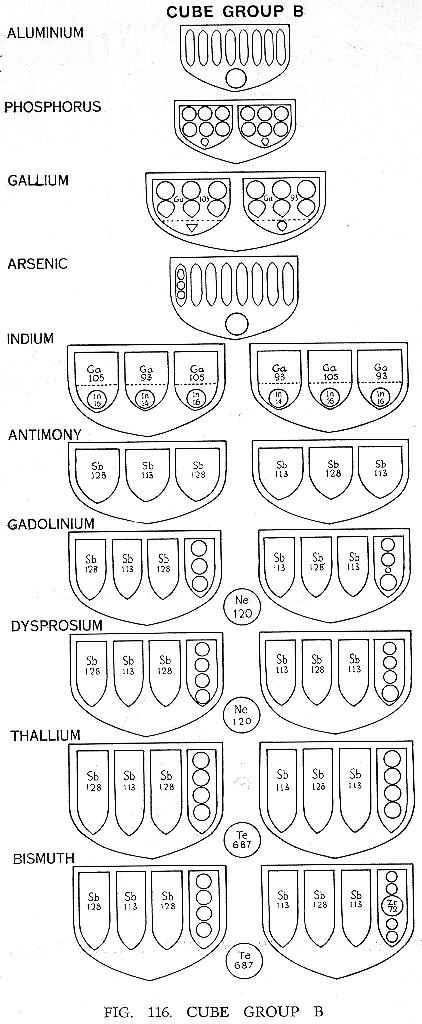FIG. 116. Cube Group B [ERRATA Fig. 116. The Indium funnel B should contain two In 14 and one In 16.]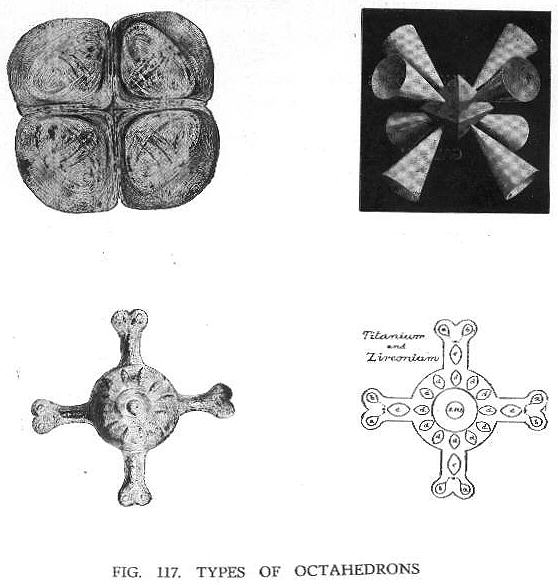FIG. 117. TYPES OF OCTAHEDRA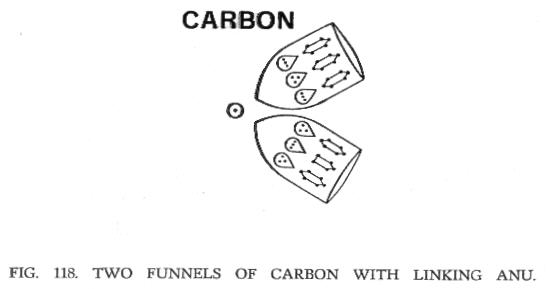FIG. 118. TWO FUNNELS OF CARBON WITH LINKING ANU.

Next: Chapter IX   The Octahedron Group A

## Acknowledgements

 TPH Twilight Archive

HTML validation by:

 W3C online validation service for HTML 4.0

spell-checked, files merged, some errors corrected,
reset in HTML 4.0 in March, April, May 2000
last revision November 2003